Next: -adic Heights Up: Non-classical Modular Forms Previous: Hilbert-Siegel Modular Forms   Contents

# Modular forms on higher rank groups

(Written by Paul Gunnells.)

Here are some problems, both computational and theoretical, about computing with cohomology of arithmetic groups in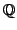-rank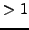. Many of these are discussed in more detail in the appendix to the book [Ste07]. I thank Avner Ash for suggesting problems (2) and (3), and for many helpful discussions.

Problem 5.2.1
1. Implement a robust user-friendly program to explore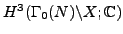, where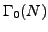is a congruence subgroup of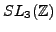, and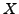is the symmetric space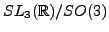. In particular your program should be able to compute a basis for the cohomology space and compute the action of the Hecke operators. One public version of such a program exists on the net at the homepage of Wilberd van der Kallen, but it's in Pascal and isn't maintained. Nevertheless it might be a good starting point.
2. Beef up your program to include local coefficient systems.
3. Beef it up even more to include integral and torsion coefficients.
4. Distribute your tool to the world by incorporating it into SAGE [SJ05].

Problem 5.2.2
1. Let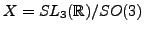and let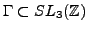be a congruence subgroup. Investigate the cohomology of the boundary of the Borel-Serre compactification of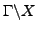with coefficients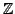or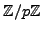.
2. Use the algorithm in [Gun00] to compute the Hecke action on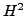of the boundary.
3. Extend these computations to other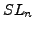s.

Problem 5.2.3   Study the space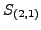of generalized modular symbols for congruence subgroups of(cf. [Ste07, A.6.8]) in a variety of ways:
1. Compute these spaces, perhaps using the tool you developed in Problem (1). Get data.
2. Develop a combinatorial model for these spaces analogous to the modular symbol model for the top degree cohomology. (This might involve generalizing the Tits building in a nontrivial way.)
3. Explain how to compute the Hecke action on the space spanned by the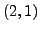modular symbols using your model.
4. Investigate generalized modular symbols on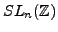for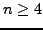.
5. There's no reason to stop with. Investigate generalized modular symbols computationally on subgroups of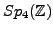, perhaps using the retract of MacPherson-McConnell [MM93], cf. [Ste07, A.6.4].

Problem 5.2.4
1. Generalize the algorithm in [Gun00], which computes the Hecke action on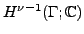(at least for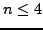), to deeper cohomology groups. Run tests similar to those in [Gun00] to tweak and polish your algorithm.

2. Perform computations with your algorithm. A natural place to start is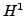of subgroups ofwithcoefficients. By work of Ash [Ash92], such cohomology classes are connected to abelian Galois representations. Another check is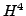of subgroups of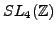. The results there could be compared to [AGM02]. For new results, you could apply your algorithm to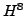of subgroups of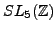.

Problem 5.2.5
1. Extend the algorithm of [Gun00] to the cohomology of subgroups of.
2. (G.Harder [Har]) Use your algorithm to investigate congruences between Siegel modular forms and elliptic modular forms.

Problem 5.2.6
1. Investigate the connections between the different notions of perfect quadratic forms in the literature (cf. [Ste07, A.6.2]).
2. Can the computational data from the work of Baeza-Coulangeon-Icaza-O'Ryan [BCIO01] be used to construct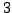(real) dimensional deformation retracts of Hilbert modular varieties that can be used to compute cohomology?
3. If not deformation retracts, can you use the data to construct cell complexes with actions of Hilbert modular groups that can then be used to compute cohomology?

Problem 5.2.7   Study the Vorono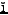polyhedron for complex quadratic fields for-ranks(cf. [Sta79]).

Problem 5.2.8
1. Study the geometry and combinatorics of the retract for[MM93,MM89]. Use the retract to compute cohomology of subgroups ofwith various coefficients.
2. Can you characterize the sets of vectors that parameterize cells in the retract, analogous to Vorono's characterization for theretract?
3. Can you define a retract for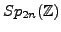?Next: -adic Heights Up: Non-classical Modular Forms Previous: Hilbert-Siegel Modular Forms   Contents
William Stein 2006-10-20